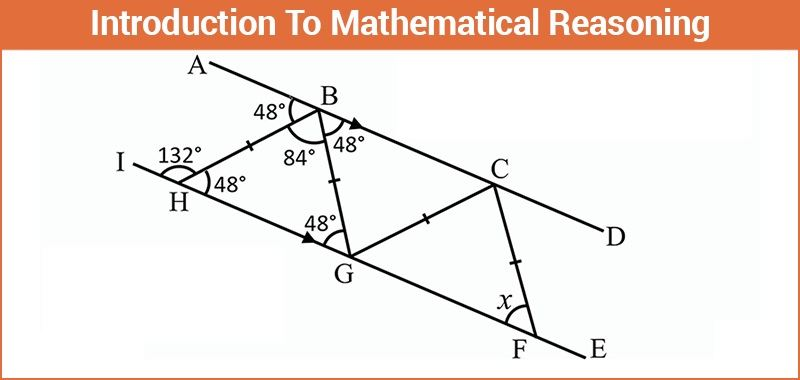Principle of Mathematical Reasoning

Since the dawn of human civilization, there has been a continuous growth in terms of innovations and technologies. Have you ever wondered why humans are the only one to have excelled everywhere? The answer is quite simple, it is because of our ability to think and put our brains to use. This power of thinking imparted us with an unending thirst for better survival methods and knowledge. As far as Mathematics is considered, this ability of humans to reason helped us to solve huge mathematical hurdles and quest for knowledge made us to reason. Pertaining to Mathematics, the reasoning can be of two types, the first one being inductive and the other one being deductive. In inductive method of reasoning, the validity of the statement is checked by certain set of rules and then it is generalized. The principle of mathematical Induction uses the concept of inductive reasoning.

In deductive reasoning,we apply the rules of a general case to a given statement and make it true for particular statements. This is actually opposite of principle of induction.Let us now discuss more about reasoning. The foremost requirement for deductive reasoning is the presence of a statement which is to be reasoned upon.

Consider the following set of statements:

i) Sun rises in the east .

ii) New Delhi is a country.

iii) Red rose is more beautiful than yellow rose.

When we read the first statement we can straightaway say that the first statement is definitely true and the second one is definitely false. As far as the third statement is considered it may depend upon perceptions of different people. Hence, it can be true for some people and at the same time false for others. But such ambiguous statements are not acceptable for reasoning in mathematics.

Thus a sentence is only acceptable mathematically when it is either true or false but not both at the same time.

So now let us discuss in terms of mathematics whether the following statements are mathematically acceptable or not.

1) The sum of three natural numbers x,y and z is always negative.

This statement is acceptable. It can never be true because all natural numbers are greater than zero and therefore the sum of positive numbers can never be negative.

2) The product of three real numbers x,y and z is always zero.

In this given statement we cannot figure out if the statement is true or false. Such a sentence is not mathematically acceptable for reasoning.

Example:

a: A circle with infinite radius is a line

b: A circle with zero radius is a point

Since a is true and b is also true then a and b is also true

For two given statements a or b to be true, show that either a is true or prove that b is true i.e. if any one of the statements is true then a or b is also true.

Example

a: 8 is multiple of 64

b: 8 is a factor of 62

Since one of the given statements i.e. a is true therefore a or b is true.

According to mathematical reasoning, if we encounter an if-then statement i.e. if a then b, then by proving that a is true b can be proved to be true or if we prove that b is false then a is also false.

If we encounter a statement which says a if and only if b then we can reason such a statement by showing that a is true and then b is also true and if b is true then a is definitely true.

Contradiction Method: In this method, we assume that the given statement is false and then try to prove the assumption wrong.

Example

a: The derivative of $y = 9x^{2} + \sin x$ w.r.t x is $18x + \cos x$.

For proving the validity of this statement, let us say that dy/dx ≠ 18x + cos x. We know that the derivative of $x^{n}$ is given by $n.x^{n-1}$ therefore, the derivative of $9x^{2}$ is 18x and the derivative of sin x is given by cos x.

Also,

$\frac{\mathrm{d} }{\mathrm{d} x} \left ( f(x) + g(x) \right ) = \frac{\mathrm{d} f(x)}{\mathrm{d} x} + \frac{\mathrm{d} g(x)}{\mathrm{d} x}$

Therefore, $\frac{\mathrm{d} }{\mathrm{d} x} \left ( 9x^{2} + \sin x \right ) = 18x + \cos x$<

Hence, our assumption is wrong and the statement a is a valid statement.

Another method for proving validity is to use a counter statement i.e. giving a statement or an example where the given statement is not valid.

Example

a: If a is the prime number then a is always odd.

To show that the given statement is false we will try to find a counter statement for this. We know that 2 is a prime number i.e. it is divisible by only itself and 1 and also 2 is the smallest even number. Therefore, we can say that 2 is a prime number which is even. Hence, we can say that the statement a is not true for all prime numbers, therefore, the given statement is not valid.

We have now seen the basic thought behind mathematical reasoning. To learn more about this please log on to www.byjus.com